## a gas with a volume of 20.0l at a pressure of 275 kpa is allowed to expand to a volume of 35.0l. what is the pressure in the container if th

Question

a gas with a volume of 20.0l at a pressure of 275 kpa is allowed to expand to a volume of 35.0l. what is the pressure in the container if the temperature remains constant?

1. 2.54 kPa

2. 157 kPa

3. 481 kPa

pls help ;(

in progress 0
3 months 2021-07-29T14:20:09+00:00 1 Answers 5 views 0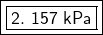Explanation:

Since temperature remains constant, the only variables that change are volume and pressure. Therefore, we are using Boyle’s Law. This states that the pressure is inversely proportional to the volume. The formula is: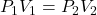We know the gas starts with a volume of 20.0 liters at a pressure of 275 kPa. We can substitute these values into the left side of the formula.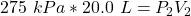We know the gas expands to a volume of 35.0 Liters, but we do not know the pressure.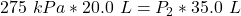Since we are solving for the new pressure, we must isolate the variable P₂. It is being multiplied by 35.0 Liters and the inverse of multiplication is division. Divide both sides by 35.0 L.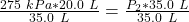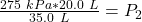The units of liters cancel.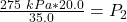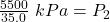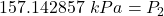The original measurements of pressure and volume have 3 significant figures, so our answer must have the same. For the number we calculated, that is the ones place.

The 1 in the tenths place (157.142857) tells us to leave the 7 in the ones place.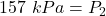If the gas expanded to a volume of 35.0 liters while the temperature remained constant, the pressure in container was approximately 157 kilopascals.